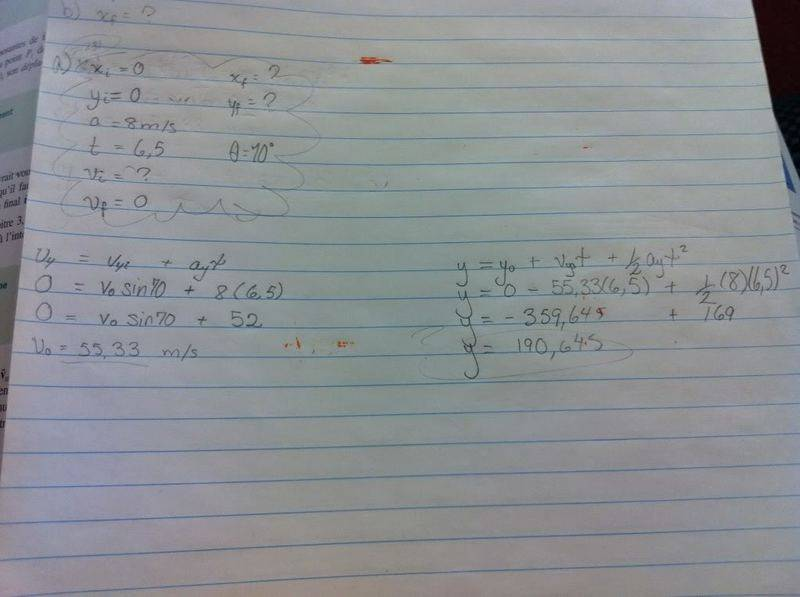# A rocket rises straight at a 70 degree angle then falls.using its acceleration,find

MissJewels

## Homework Statement

For 6.5 s, a rocket rises in a straight line oriented at 70° from the horizontal axis with a constant acceleration module of 8m/s2. Then she is goes into free fall.
Find:
a) the maximum height
b) its horizontal reach

## Homework Equations

t = ( vfv - viv)/a
d = viht + (0.5)at2
I believe?
y= yi + vyot -(0.5)gt2
x= vxot

## The Attempt at a Solution

so ive got these given infos:
t= 6,5
xi= 0
xf= ?
yi= 0
yf= ?
a= 8 m/s2

a) to find yf at vf=0

y= vo(sin(70))t -(0,5)(9,8)t2

But then, i wonder if i should replace gravity with acceleration, but seeing as were working with y, doesnt that mean i HAVE to use -9,8 as my g (or a) value? From what i understand, I only use 8m/s2 when i have to calculate wth the values of x? I'm confused. Someone explain that at least! I have a strong feeling im heading in the wrong direction with my work so far. Boost me?

b) as for this one, im guessing i have to find xf after 6,8 seconds once i find the value of v0, I'm guessing things will fall into place. But do correct me if im wrong?

## Answers and Replies

Staff Emeritus
Homework Helper

The key to this problem is that there are two parts to the motion. The acceleration is different for each part, so you have to do separate calculations for each part:

(1) The acceleration is 8 m/s2, in the same direction that the rocket is traveling. So the rocket moves in a 70°-from-horizontal straight line, starting from rest, for 6.5 s. You first need to figure out how far something moves in a straight line with that acceleration, and then figure out the x and y coordinates given that it was travelling at 70°.

(2) The rocket is in free fall, i.e. standard projectile motion with an acceleration of 9.8 m/s2 downward. The initial velocity and position are whatever you calculate for final position and velocity at the end of phase (1). This phase lasts until the rocket hits the ground.

MissJewels

The key to this problem is that there are two parts to the motion. The acceleration is different for each part, so you have to do separate calculations for each part:

(1) The acceleration is 8 m/s2, in the same direction that the rocket is traveling. So the rocket moves in a 70°-from-horizontal straight line, starting from rest, for 6.5 s. You first need to figure out how far something moves in a straight line with that acceleration, and then figure out the x and y coordinates given that it was travelling at 70°.

(2) The rocket is in free fall, i.e. standard projectile motion with an acceleration of 9.8 m/s2 downward. The initial velocity and position are whatever you calculate for final position and velocity at the end of phase (1). This phase lasts until the rocket hits the ground.

I just took a picture of my work, but heres my attempt at a) the answer is SUPPOSED to be yf=281 but im getting 190... whats wrong?Staff Emeritus